Question

The following reaction:

H2 (g) + I2 (g) ⇋ 2 HI (g)

Has an equilibrium constant of 30.5 under certain conditions. If initial concentrations of reactants and product are: [H2] = 0.100 M; [I2] = 0.100 M; and [HI] = 0.250 M, what will be the equilibrium concentration of HI?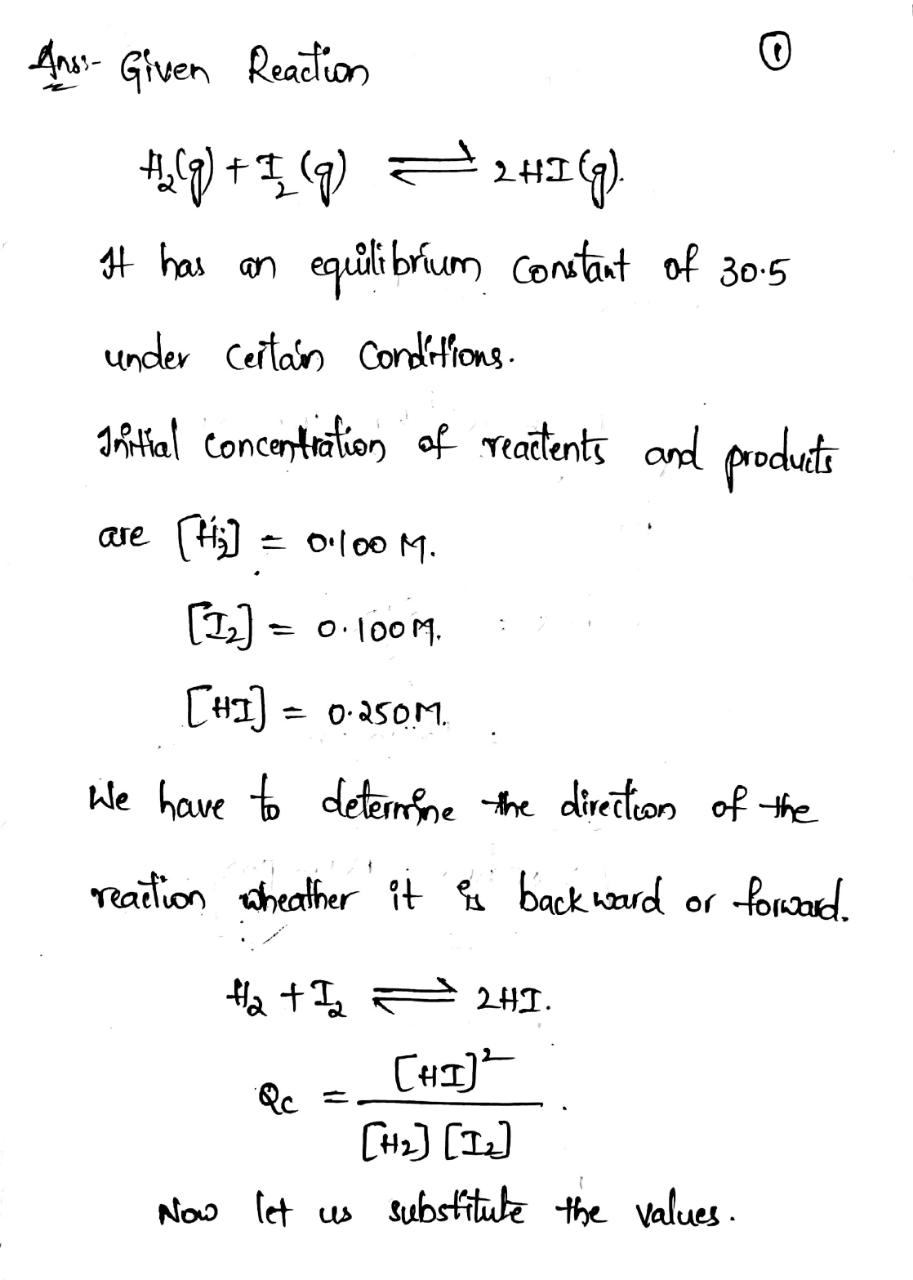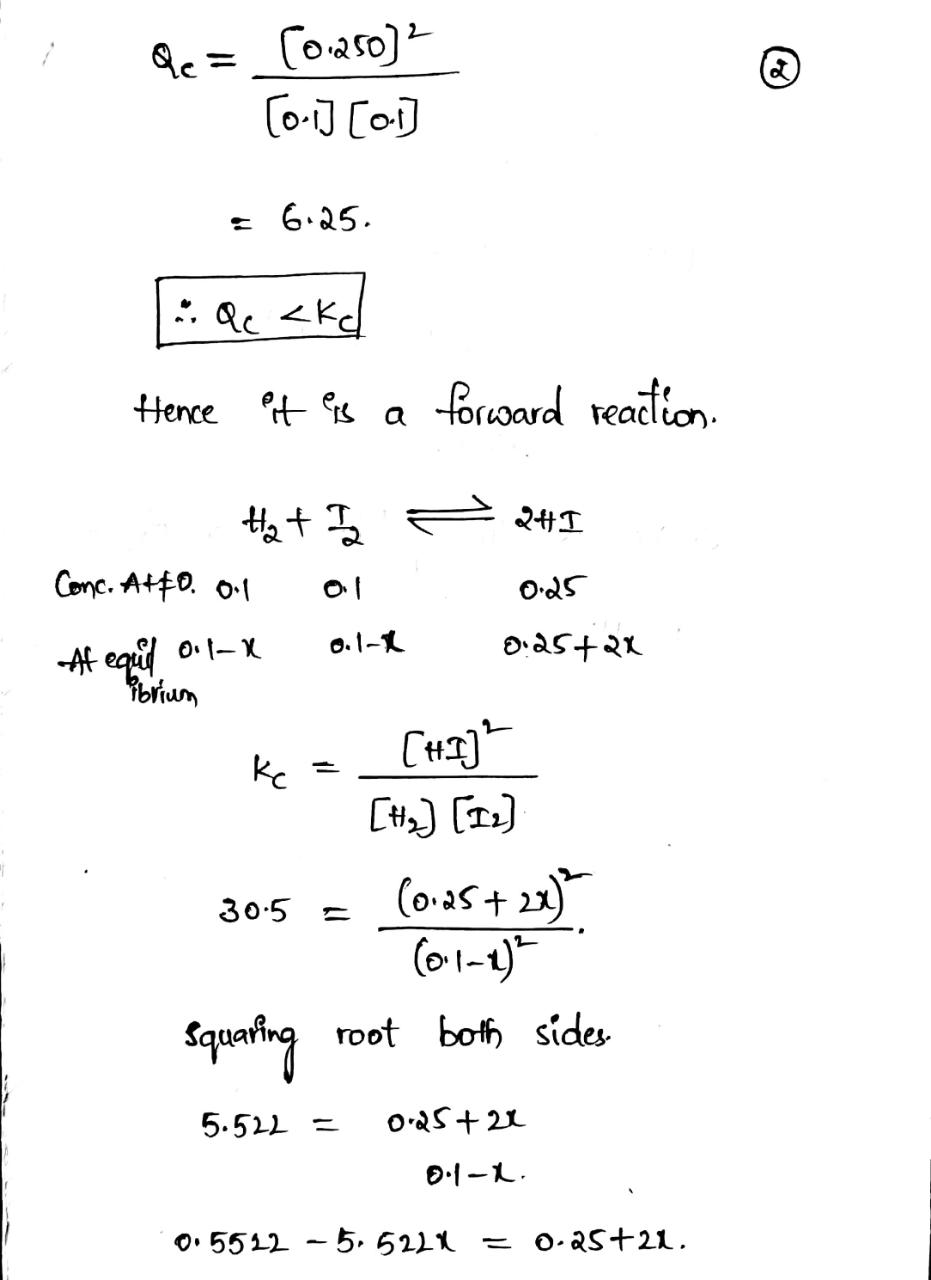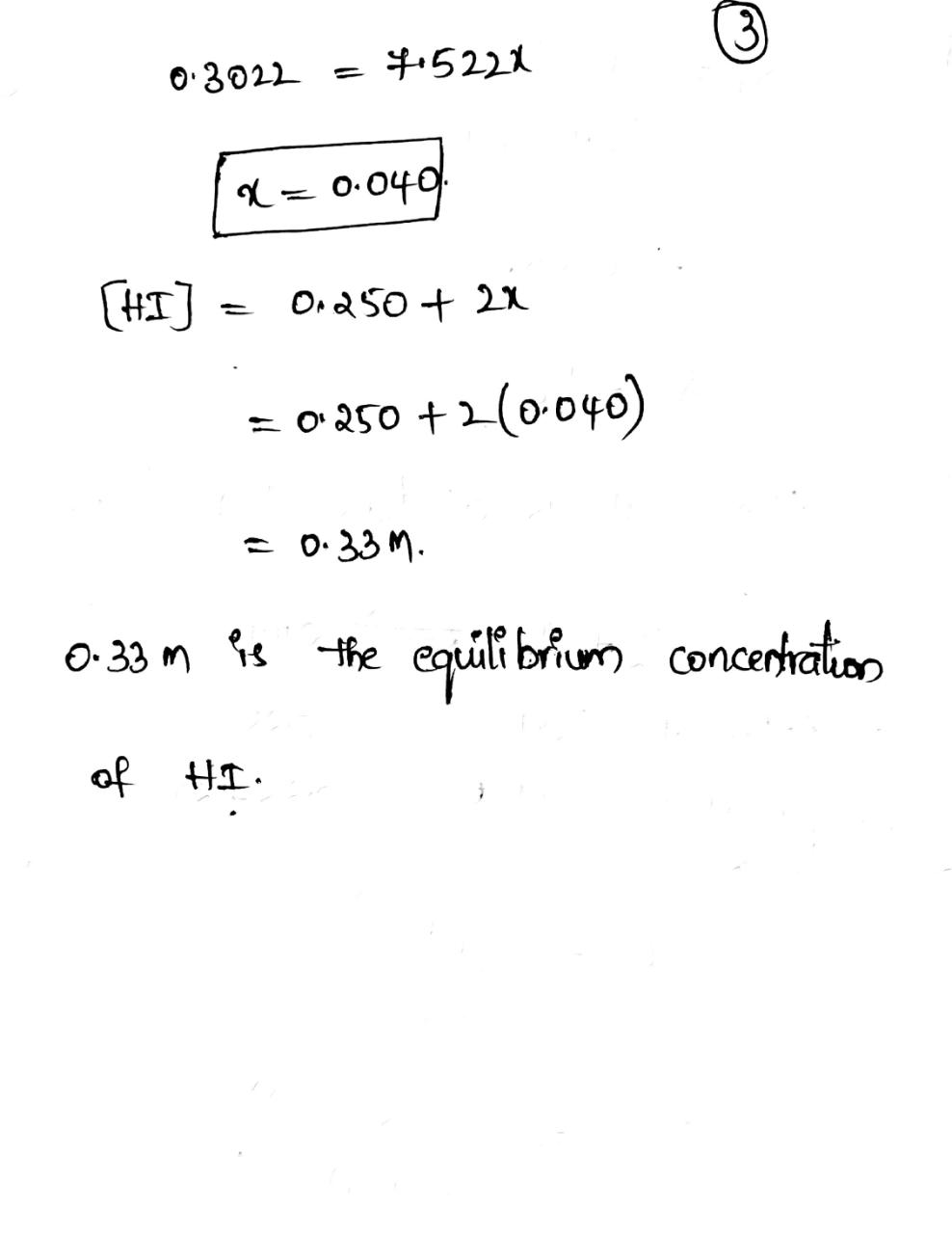#### Earn Coins

Coins can be redeemed for fabulous gifts.

Similar Homework Help Questions
• ### consider the equilibrium reaction of H2 (g) + I2 (g) <-----> 2 HI (g) it has...

consider the equilibrium reaction of H2 (g) + I2 (g) <-----> 2 HI (g) it has an equilibrium constant Kc = 54.3 at 430°C. if the initial concentration of [HI]0 = 2.000 M. what are the equilibrium concentrations?

• ### The equilibrium constant, Kc, for the following reaction is 55.6 at 698 K: H2(g) + I2(g)...

The equilibrium constant, Kc, for the following reaction is 55.6 at 698 K: H2(g) + I2(g) 2HI(g) Calculate the equilibrium concentrations of reactants and product when 0.293 moles of H2 and 0.293 moles of I2 are introduced into a 1.00 L vessel at 698 K. [H2] = M [I2] = M [HI] = M

• ### The equilibrium constant, Kc, for the following reaction is 55.6 at 698 K. H2 (g) +...

The equilibrium constant, Kc, for the following reaction is 55.6 at 698 K. H2 (g) + I2 (g) ---> 2 HI (g) Calculate the equilibrium concentrations of reactants and product when 0.301 moles of H2 and 0.301 moles of I2 are introduced into a 1.00 L vessel at 698 K. [ H2 ]   = _______ M [ I2 ]   = ________ M [ HI ]   = _______ M

• ### At 6 oC the equilibrium constant for the reaction: 2 HI(g) H2(g) + I2(g) is KP...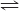At 6 oC the equilibrium constant for the reaction: 2 HI(g) H2(g) + I2(g) is KP = 2.66e-11. If the initial pressure of HI is 0.00837 atm, what are the equilibrium partial pressures of HI, H2, and I2? We were unable to transcribe this imageAt 6 °C the equilibrium constant for the reaction: 2 HI(g) = H2(g) + 12(g) is Kp = 2.66e-11. If the initial pressure of HI is 0.00837 atm, what are the equilibrium partial pressures of HI,...

• ### At 49 oC the equilibrium constant for the reaction: 2 HI(g) H2(g) + I2(g) is KP...

At 49 oC the equilibrium constant for the reaction: 2 HI(g) H2(g) + I2(g) is KP = 4.83e-11. If the initial pressure of HI is 0.00862 atm, what are the equilibrium partial pressures of HI, H2, and I2? p(HI) = p(H2) = . p(I2) = .

• ### 9. The equilibrium constant for the reaction H2(g) + I2(g) 2HI(g) has a value of 54.6 at 699K. ...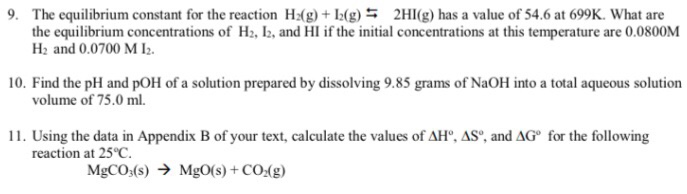9. The equilibrium constant for the reaction H2(g) + I2(g) 2HI(g) has a value of 54.6 at 699K. What are the equilibrium concentrations of H2, I2, and HI if the initial concentrations at this temperature are 0.0800M H2 and 0.0700 M I2. 10. Find the pH and pOH of a solution prepared by dissolving 9.85 grams of NaOH into a total aqueous solution volume of 75.0 ml. 11. Using the data in Appendix B of your text, calculate the values...

• ### Calculate the equilibrium concentrations of H2, I2, and HI at 700 K if the initial concentrations...

Calculate the equilibrium concentrations of H2, I2, and HI at 700 K if the initial concentrations are [H2] = 0.200 M and [I2] = 0.400 M. The equilibrium constant Kc for the reaction following reaction is 57.0 at 700 K. (Show Work) H2(g)+I2(g)<---                    ---->2HI(g)

• ### The equilibrium constant, K, for the following reaction is 1.80×10-2 at 698 K. 2HI(g) ----> H2(g)...

The equilibrium constant, K, for the following reaction is 1.80×10-2 at 698 K. 2HI(g) ----> H2(g) + I2(g) An equilibrium mixture of the three gases in a 1.00 L flask at 698 K contains 0.306 M HI, 4.10×10-2 M H2 and 4.10×10-2 M I2. What will be the concentrations of the three gases once equilibrium has been reestablished, if 0.208 mol of HI(g) is added to the flask? [HI]   = ______ M [H2]   = ______ M [I2]   = ______M

• ### The equilibrium constant, K, for the following reaction is 1.80×10-2 at 698 K. 2HI(g) H2(g) +...

The equilibrium constant, K, for the following reaction is 1.80×10-2 at 698 K. 2HI(g) H2(g) + I2(g) An equilibrium mixture of the three gases in a 1.00 L flask at 698 K contains 0.329 M HI, 4.41×10-2 M H2 and 4.41×10-2 M I2. What will be the concentrations of the three gases once equilibrium has been reestablished, if 2.54×10-2 mol of H2(g) is added to the flask? [HI] = M [H2] = M [I2] = M

• ### The equilibrium constant for the reaction: H2(g) + I2(g) <--> 2HI(g) is 54 at 700 K....

The equilibrium constant for the reaction: H2(g) + I2(g) <--> 2HI(g) is 54 at 700 K. A mixture of H2, I2 and HI, each at 0.020 M, was introduced into a container at 700 K. Which of the following is true? At equilibrium, [H2] = [I2] = [HI]. No net change occurs because the system is at equilibrium. The reaction proceeds to the left producing more H2(g) and I2(g). The reaction proceeds to the right producing more HI(g). At equilibrium,...